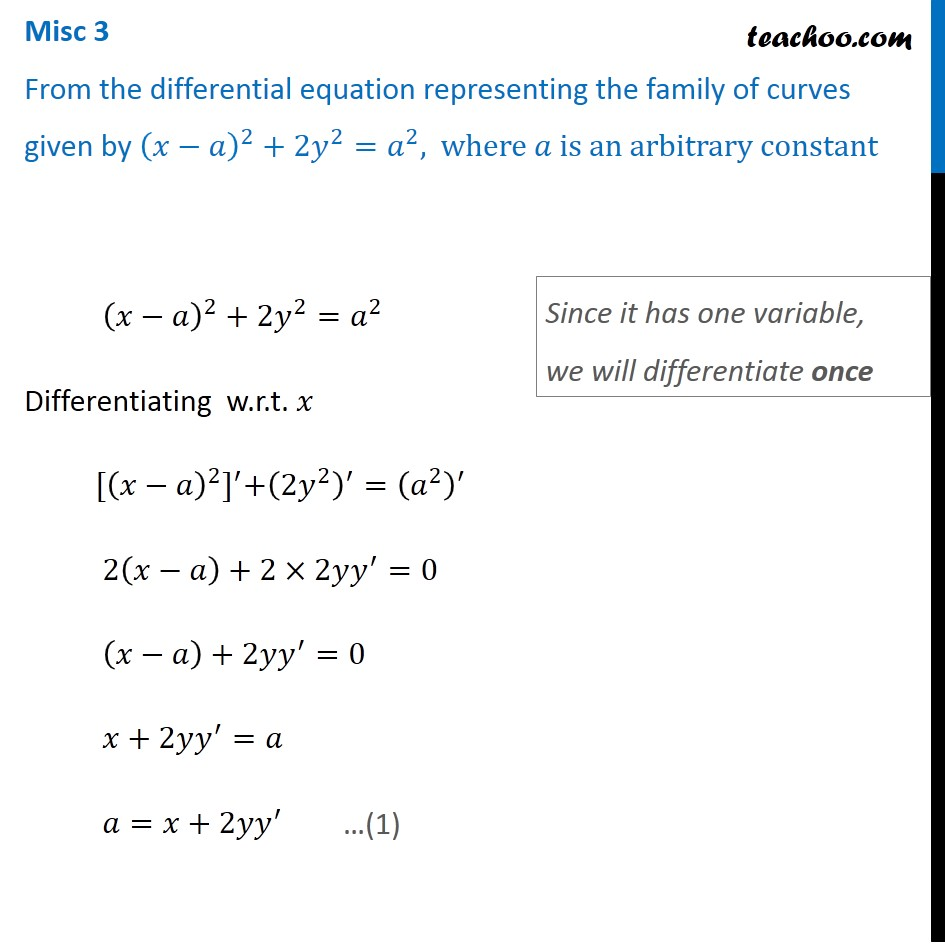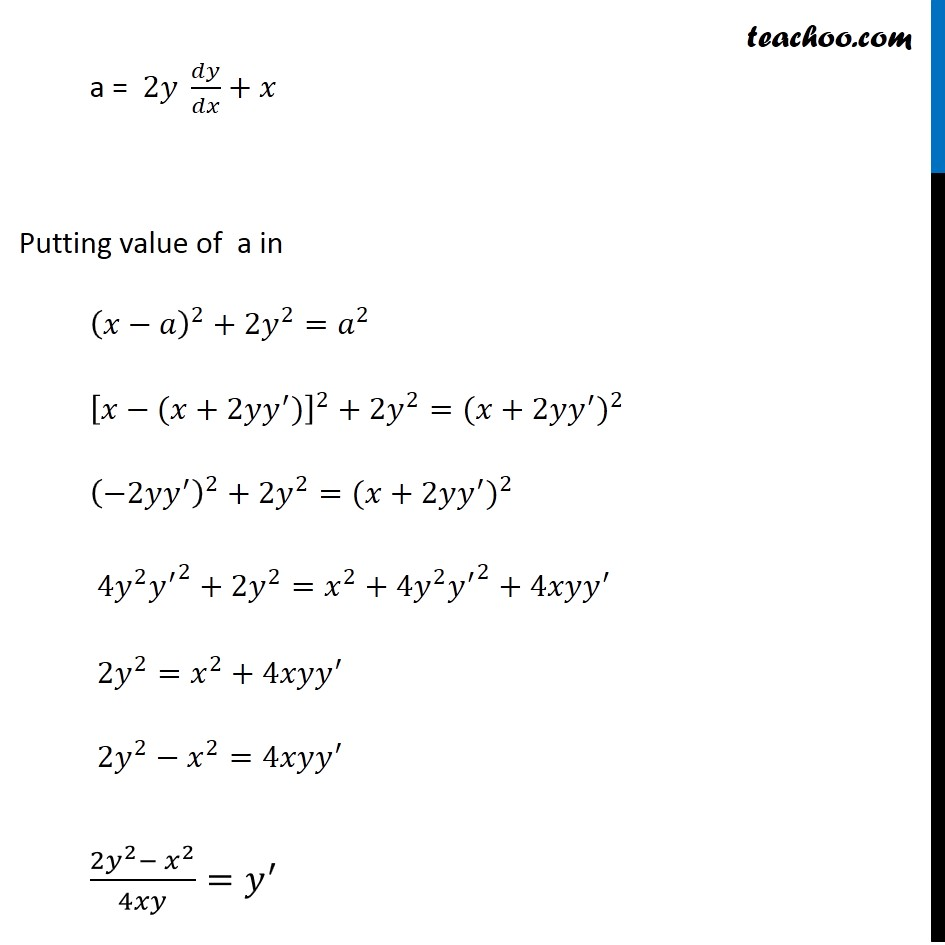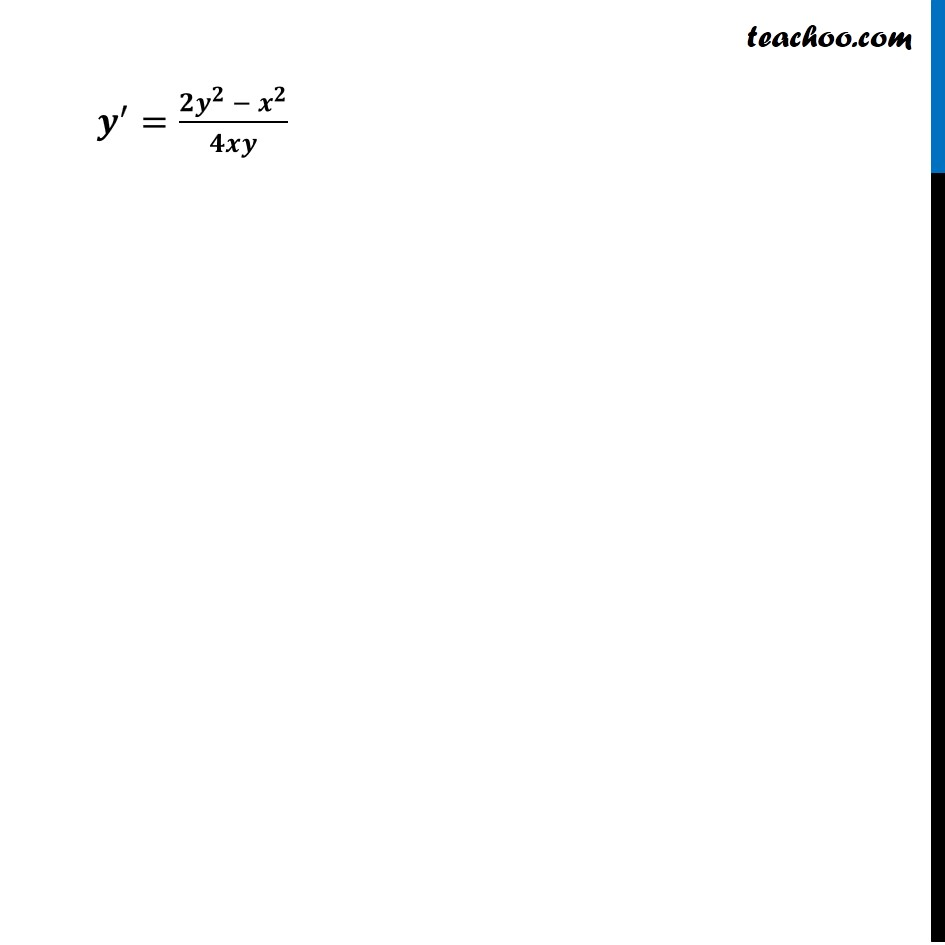Miscellaneous

Chapter 9 Class 12 Differential Equations
Serial order wiseLearn in your speed, with individual attention - Teachoo Maths 1-on-1 Class

### Transcript

Question 1 From the differential equation representing the family of curves given by (𝑥−𝑎)^2+2𝑦^2=𝑎^2, where 𝑎 is an arbitrary constant (𝑥−𝑎)^2+2𝑦^2=𝑎^2 Differentiating w.r.t. 𝑥 〖[(𝑥−𝑎)^2]〗^′+(2𝑦^2 )^′=(𝑎^2 )^′ 2(𝑥−𝑎)+2×2𝑦𝑦^′=0 (𝑥−𝑎)+2𝑦𝑦^′=0 𝑥+2𝑦𝑦^′=𝑎 𝑎=𝑥+2〖𝑦𝑦〗^′ Since it has one variable, we will differentiate once a = 2𝑦 𝑑𝑦/𝑑𝑥+𝑥 Putting value of a in (𝑥−𝑎)^2+2𝑦^2=𝑎^2 [𝑥−(𝑥+2𝑦𝑦^′)]^2+2𝑦^2=〖(𝑥+2𝑦𝑦^′)〗^2 (−2𝑦𝑦^′ )^2+2𝑦^2=〖(𝑥+2𝑦𝑦^′)〗^2 4𝑦^2 〖𝑦^′〗^2+2𝑦^2=𝑥^2+4𝑦^2 〖𝑦^′〗^2+4𝑥𝑦𝑦^′ 2𝑦^2=𝑥^2+4𝑥𝑦𝑦^′ 2𝑦^2−𝑥^2=4𝑥𝑦𝑦^′ (2𝑦^2− 𝑥^2)/4𝑥𝑦=𝑦^′ 𝒚^′=(𝟐𝒚^𝟐 − 𝒙^𝟐)/𝟒𝒙𝒚